9 MCQ on macroeconomics

# 9 MCQ on macroeconomics - Commerce

Needed a Test for microeconomics?

Ref: https://edurev.in/question/761644/Needed-a-Test-for-microeconomics-Related-Economics-for-Class-12-XII-CBSE-and-NCERT-Curriculum

 1 Crore+ students have signed up on EduRev. Have you?
• 1.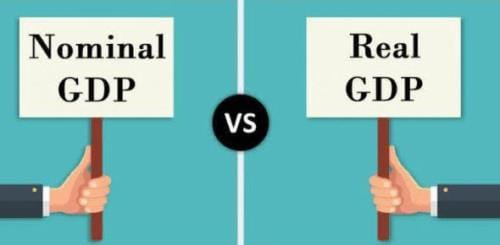Diffrence between Real and Nominal GDP is
• A.

Measured by excluding some of the sectors

• B.

That real GDP is always smaller than Nominal GDP

• C.

Change in price level from base year to current year

• D.

None of the above.

• 2.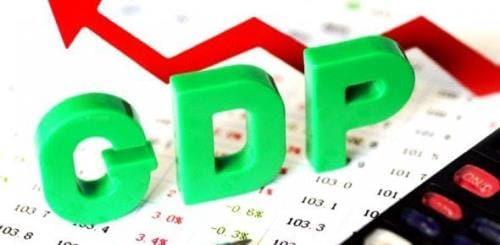GDP is  market value of all the final goods
• A.

Produced domestically

• B.

Produced by domestic factors of production

• C.

Produced by all factors of production

• D.

All of the above

• 3.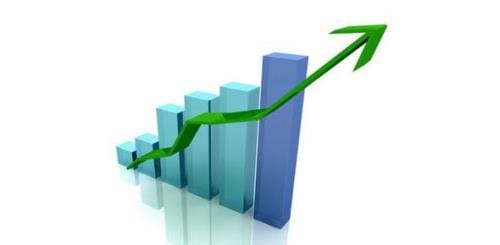When comparing nation's economic position with other one should see its
• A.

GDP

• B.

Per Capita GDP

• C.

Currency in circulation

• D.

None of the above

• 4.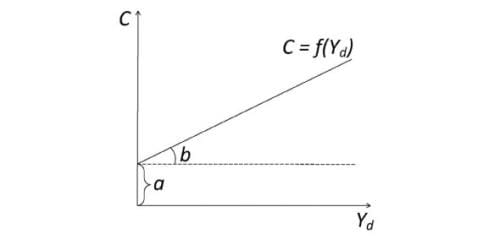Verticle intercept of consumption function indicates
• A.

Average propensity to consume

• B.

Consumption at zero level of income

• C.

Both of the above

• D.

None of the above

• 5.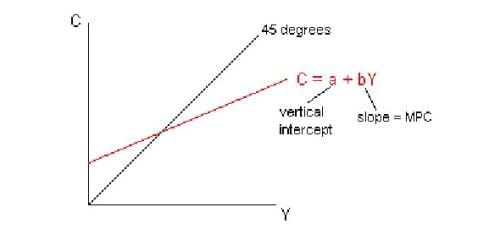In consumption function c=a by
• A.

B is intercept

• B.

Slope of the function

• C.

MPC

• D.

A & C

• 6.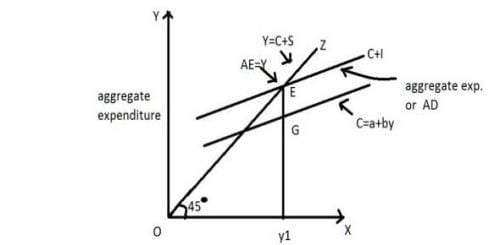In Keynesian Framework Income is measured along
• A.

45 degree line

• B.

Verticle axis

• C.

Horizontal axis

• D.

All are correct

• 7.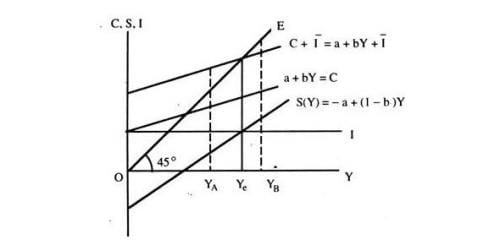If investment is exogenous to the Income determination model it can be shown as
• A.

Verticle line

• B.

Horizontal line

• C.

All of the above

• D.

None of the above

• 8.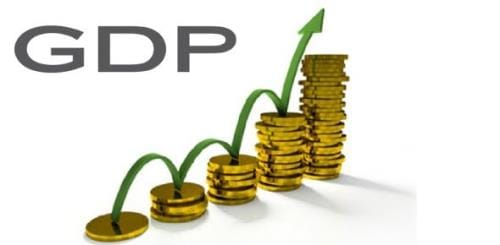In closed economy GDP equals
• A.

C I X-N

• B.

C I

• C.

C I G

• D.

All of the above

• 9.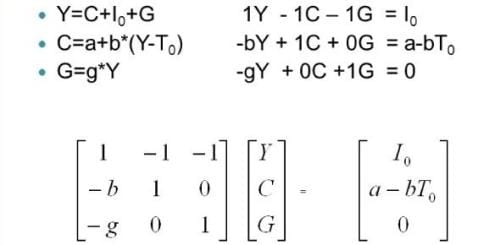In the model Y=C I G and C=a by Where b=0.8 , the expenditure multplier is
• A.

1

• B.

4

• C.

5

• D.

None of the above

The document 9 MCQ on macroeconomics - Commerce is a part of Commerce category.
All you need of Commerce at this link: Commerce

Track your progress, build streaks, highlight & save important lessons and more!

,

,

,

,

,

,

,

,

,

,

,

,

,

,

,

,

,

,

,

,

,

;